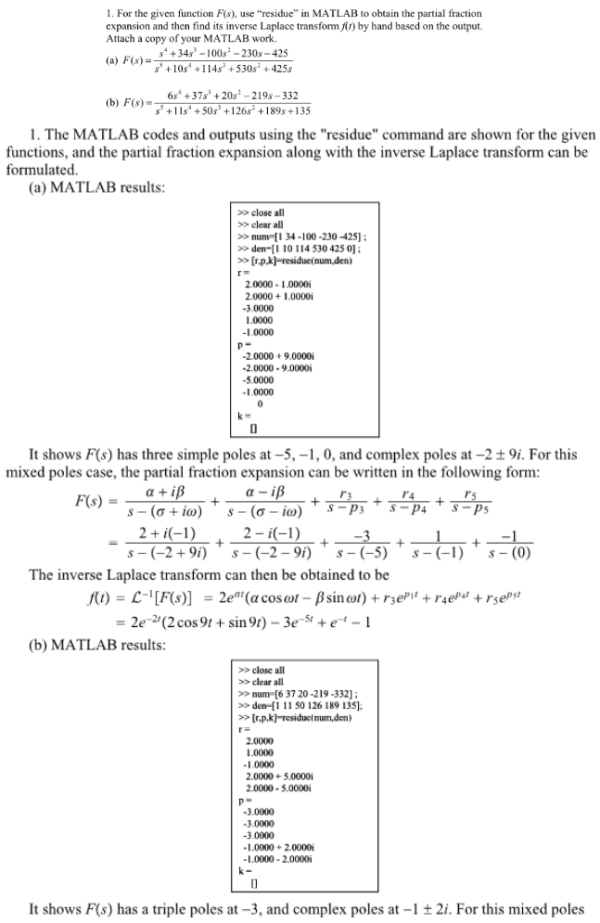For the given function F(s), use residue in MATLAB to obtain the partial fraction expansion and then find its inverse Laplace transform f(t) by hand based on the output.For the given function F(s), use residue in MATLAB to obtain the partial fraction expansion and then find its inverse Laplace transform f(t) by hand based on the output.

System Dynamics Page 2 dynamics dynamics dynamics dynamics dynamics dynamics dynamics System dynamics Page 3